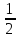SHARE
HELP

To start with, Sr2Jr’s first step is to reduce the expenses related to education. To achieve this goal Sr2Jr organized the textbook’s question and answers. Sr2Jr is community based and need your support to fill the question and answers. The question and answers posted will be available free of cost to all.Authors:
Walter Savitch ,kenrick Mock
Chapter:
C++ Basics
Exercise:
Programming Projects
Question:10 | ISBN:9780132846813 | Edition: 5

###Question

Write a program that allows the user to enter a time in seconds and then outputs how far an object would drop if it is in freefall for that length of time. Assume no friction or resistance from air and a constant acceleration of 32 feet per second due to gravity. Use the equation

Distance =* acceleration * time2

###Answer

``````#include<iostream>
using namespace std;

#define ACCELERATION 32

int main()
{
//Variables
int time;
double distance;

//Input from the user
cout<<" Enter time(Seconds):";
cin>>time;

//Compute distance using the formula:
//distance=(acceleration*time^2)/2
distance = ((ACCELERATION * time *time)/2);

//Display result
cout<<"Traveled distance:" << distance <<"\n";
}``````

Result output:

``````Enter time(Seconds):60
Traveled distance  :57600``````

### Discussions

Post the discussion to improve the above solution.